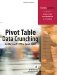You need to master a few simple techniques in order to write efficient VBA code. These techniques will help you make the jump to writing effective code.

#### Write Code to Handle Any Size Data Range

The macro recorder will hard-code that your data is in a range, such as A1:K41550. Although this will work for today's dataset, it may not work as you get new datasets. Write code that can deal with different size datasets.

The macro recorder will use syntax such as Range("H12") to refer to a cell. However, it is more flexible to use Cells(12, 8) to refer to the cell in row 12, column 8. Similarly, the macro recorder will refer to a rectangular range as Range("A1:K41550"). However, it is more flexible to use the Cells syntax to refer to the upper-left corner of the range and then use the Resize() syntax to refer to the number of rows and columns in the range. The equivalent way to describe the preceding range is Cells(1, 1).Resize(41550,11).

This is more flexible because you can replace any of the numbers with a variable.

In the Excel user interface, you can use the End key on the keyboard to jump to the end of a range of data. If you move the cellpointer to the final row on the worksheet and press the End key followed by the up-arrow key, the cell pointer will jump to the last row with data. The equivalent of doing this in VBA is to use the following code:

` Range("A65536").End(xlUp).Select `

You don't need to select this cellyou just need to find the row number that contains the last row. The following code will locate this row and save the row number to a variable named FinalRow:

` FinalRow = Range("A65536").End(xlUp).Row `

There is nothing magic about the variable name FinalRow. You could call this variable something such as x or y, or even your dog's name. However, because VBA allows you to use meaningful variable names, you should use something such as FinalRow to describe the final row.

NOTE

Excel has offered 65,536 rows for eight years and 256 columns for 20 years. Some predict that Microsoft will finally offer more rows and columns in Excel 2006. To make your code flexible enough to handle newer versions of Excel, you can use Application.Rows.Count to learn the total number of rows in this version of Excel. The preceding code could then be generalized like so: FinalRow = Cells(Application.Rows.Count, 1).End(xlUp).Row

It is also possible to find the final column in a dataset. If you are relatively sure that the dataset will begin in row 1, you can use the End key in combination with the left-arrow key to jump from IV1 to the last column with data. To generalize for the possibility that Excel 2006 includes more columns, you can use the following code:

` FinalCol = Cells(1, Application.Columns.Count).End(xlToLeft).Column `

## End+Down Versus End+Up

You might be tempted to find the final row by starting in cell A1 and using the End key in conjunction with the down-arrow key. Avoid this. Data coming from another system is imperfect. If your program will import 50,000 rows from a legacy computer system every day for the next five years, a day will come when someone manages to key a null value into the dataset. This will cause a blank cell or even a blank row to appear in the middle of your dataset. Using Range("A1").End(xlDown) will stop prematurely at the blank cell instead of including all your data. This blank cell will cause that day's report to miss thousands of rows of data, a potential disaster that will call into question the credibility of your report. Take the extra step of starting at the last row in the worksheet in order to greatly reduce the risk of problems.

#### Use Super-VariablesObject Variables

In typical programming languages, a variable holds a single value. You might use x = 4 to assign a value of 4 to the variable x.

Think about a single cell in Excel. There are many properties that describe a cell. A cell might contain a value such as 4, but the cell also has a font size, font color, a row, a column, possibly a formula, possibly a comment, a list of precedents, and more. It is possible in VBA to create a super-variable that contains all the information about a cell or about any object. A statement to create a typical variable such as x = Range("A1") will assign the current value of A1 to the variable x. However, use the Set keyword to create an object variable:

` Set x = Range("A1") `

You've now created a super-variable that contains all the properties of the cell. Instead of having a variable with only one value, you have a variable where you can access the value of many properties associated with the variable. You can reference x.Formula to learn the formula in A1, or x.Font.ColorIndex to learn the color of the cell.Pivot Table Data Crunching for Microsoft Office Excel 2007
ISBN: 0789736012
EAN: 2147483647
Year: 2003
Pages: 140

Similar book on Amazon